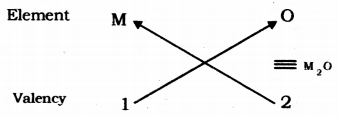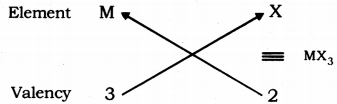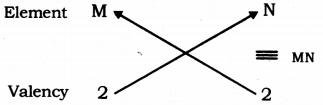# Based on the group valency of elements. State the formula for the following giving justification for each:

Based on the group valency of elements. State the formula for the following giving justification for each:
(i) Oxides of Ist group elements,
(ii) Halides of the elements of group 13, and
(iii) Compounds formed when an element of group 2 combines with an element of group 16.

(i) Valency of group 1 elements: 1
Valency of oxygen: 2
Oxides of group 1 elements:Formula of the oxides of group 1 is M20, where M is the group 1 element and O is oxygen.

(ii) Valency of group 13 elements: 3
Valency of halogens : 1
Halides of group 13 elements:Formula of the halides of group 1 is MX3, where, M is the group 13 element and X is halogen.

(iii) Valency of group 2 elements: 2
Valency of group 16 elements: 2
Compounds of group 2 and group 16 elements:Formula of the compounds of group 2 and 16 is MN, where, M is the group 2 element and N is the group 16 element.